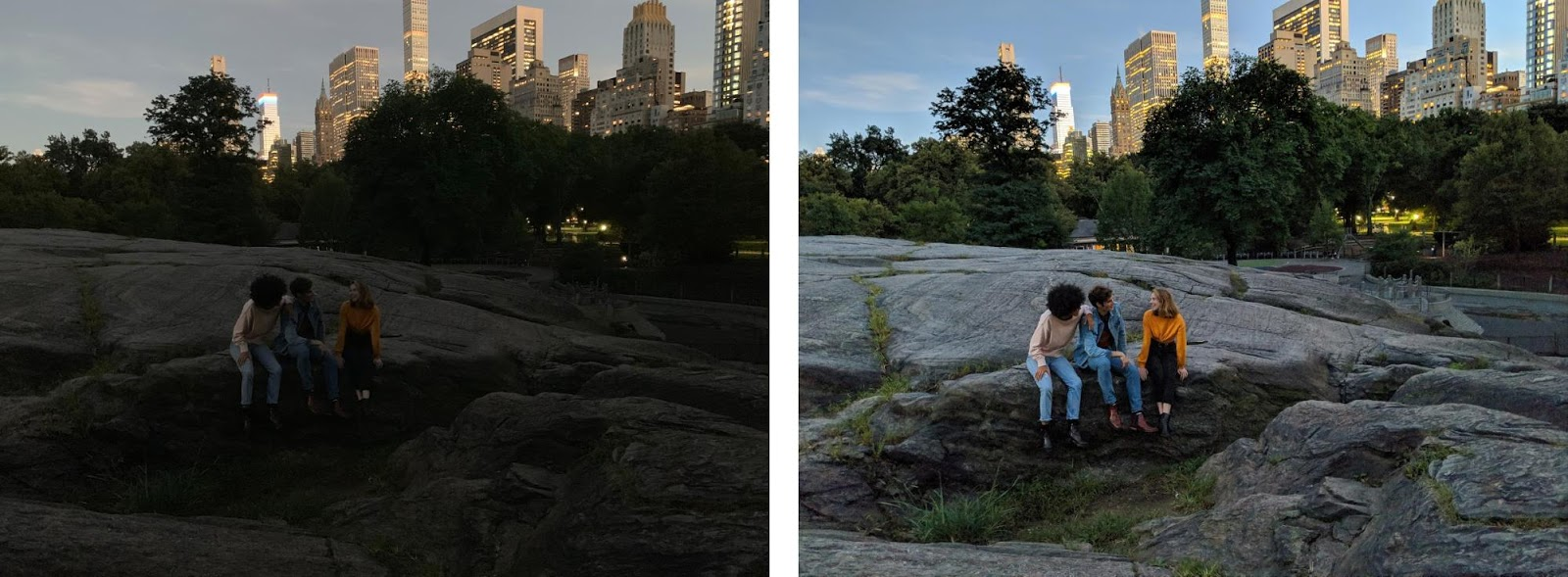# Night Sight

In this problem we explore how to use probability theory to take photos in the dark. Digital cameras have a sensor that capture photons over the duration of a photo shot to produce pictures. However, these sensors are subject to “shot noise” which are random fluctuations in the amount of photons that hit the lens. In the scope of this problem, we only consider a single pixel. The arrival of shot noise photons on a surface is independent with constant rate.Left: photo captured using a standard photo. Right: the same photo using a shot burst .

For shot noise, standard deviation is what matters! Why? Because if the camera can compute the expected amount of noise, it can simply subtract it out. But the fluctuations around the mean (measured as standard deviation) lead to changes in measurement that the camera can't simply subtract out.

### Part 1: A Standard Photo

First lets calculate the amount of noise if we take a photo the standard way. If the time duration of a photo shot is 1000 𝜇s, what is the standard deviation of the amount of photons captured by the pixel during a single photo? Note that shot noise photons land on a particular pixel at a rate of 10 photons per microsecond (𝜇s).

Noise in a standard photo: As you may have guessed, because photos hit the camera at a constant rate, and independent of one another, the number of shot noise photos hitting any pixel is modelled as a Poisson! For the given rate of noise, let $X$ be the amount of shot noise photos that hit the pixel: $$X \sim \Poi(\lambda = 10,000).$$ Note that 10,000 is the average number of photons that hit in 1000𝜇s (duration in microseconds multiplied by photons per microsecond). The standard of a Poisson is simply equal to the square root of its parameter, $\sqrt{\lambda}$. Thus the standard deviation of the shot noise photons captured is 100 (quite high).

### Part 2: A Shutter Shot

To mitigate shot noise, Stanford graduates realized that you can take a shutter shot (many camera shots in quick succession) and sum the number of photons captured. Because of limitations in cell phone cameras, the largest number of photos a camera can take in 1000$\mu$s is 15 photos, each with a duration of 66$\mu$s. What is the standard deviation of shot noise if we average the photons across a shutter shot of 15 photos?

Noise with a shutter shot:

Let $Y$ be the average quantity of shot noise photons across the 15 photos, captured by the single pixel. We want to calculate the $\text{Var}(Y)$. Specifically, $Y = \frac{1}{15}\sum_{i=1}^{15} X_i$ where $X_i$ is the amount of shot noise photons in the ith photo. Similar to the previous part: $$X_i \sim \Poi(\lambda = 66 \cdot 10)$$ and since $X_i$ is a Poisson, $\E[X_i] = 660$ and $\Var(X_i) = 660$.

Since $Y$ is the average of IID random variables, the Central Limit Theorem will kick in. Moreover, by the CLT rule $Y$ will have variance equal to ${1}/{n}\cdot \Var(X_i)$. \begin{align*} \Var(Y) &= {1}/{n}\cdot \Var(X_i) \\ &= 1/15 \cdot 660 = 44 \end{align*} The standard deviation will then be the square root of this variance $\Std(Y) = \sqrt{44}$ which is approximately 6.6. That is a huge reduction in shot noise!

Problem by Will Song and Chris Piech. Night Sight by Google.